# Solving the Multiarmed Bandit problem with JavaScript

## 1. Formulation of the Multiarmed Bandit Problem

Consider the following problem: A gambler enters a casino withslot machines. The probability to receive a rewardfor each slot machine follows different, unknown probabilities, e.g. we are facing a set of unknown distributionswith associated expected valuesand variances.

In each turnthe gambler can play the lever of one slot machineand observes the associated reward. The objective is now with which strategy the gambler should play to maximize his earning ( or minimizing his losses in case he has to pay a fee to pull a lever 😉 ).

If the distributions are known, then one would simply play all the times at the machine with the highest probability of winning. The average reward following this strategy will then be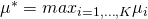.

Since the probabilities are unknown, a naive solution would for example to play several times, say, at the fist machine, then several times at the second and so on to get a decent estimator idea which machine is the best one and then keep playing. However there are certain drawbacks with this approach, first of all one will play at leasttimes at an inferior machine and secondly one can end up choosing the wrong machine in the end. The chance ending up with the wrong machine can be decreased by raising the number of turn played at each machine, but then one will play even more often at a inferior machine. To measure the performance of a certain strategy we introduce the concept of total expected regret, defined bystates that regret grows at least logarithmically. Therefore an algorithm is said to solve the multi-armed bandit problem if it can match this lower bound such that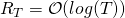. For the naive solutions we thus can derive that:

Play each machine with an uniform random propability: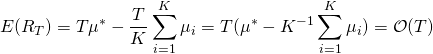Play each machine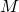times, play infinitely the machine giving the highest payoff:whereis the average winning of machineat time.

In Blog post we will discuss the Upper Confidence Bounds (UCB1) algorithm proposed by  . UCB1 is the simplest algorithm out of the UCB family. The idea of the algorithm is to initially try each lever once. Record the reward gained from each machine as well as the times the machine has been played. At each turnselect the machinewhich depends on the average winning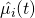as well as the numberthe machine has been played, and the trial number.
 show that for UCB1Hence UCB1 achieves the optimal regret and is said to solve the multi-armed bandit problem.

An advantage of UCB1 is that the algorithm is easy to implement. In the following we present an implementation of UCB1 using JavaScript. An advantage of JavaScript is that is a functional programming language. This means that we can pass functions as arguments of functions. In terms of the multi armed bandit algorithm this allows us to pass entire sample functions to the algorithm.

  /*
--Bandit.js
-This Program computes an optimal lever and average return of the multiarmed bandit problem
--Input
f=[f_1(x),...,f_K(x)]       -An Array of size K containing functions given a certain reward r eg. binomial or a normal distribution with different means
T                           -Runs to retrieve the decision (the first, t or  N  will terminate the algorithm)
--Output
sum \mu                     -the average revenue
x_out
--Heiko Wagner 2019
*/

bandit = function(f, T) {
//pull each lever once
var x_out = f.map((x) => [x(), 1])
var a;
for (var t = 0; t < T; t++) {
//determine the position with the highest value
var j = x_out.map((x) => x / x + Math.sqrt((2 * Math.log(t)) / x))
a = j.reduce((iMax, x, i, arr) => x > arr[iMax] ? i : iMax, 0)
//pull maximum lever
x_out[a] = [x_out[a] + f[a](), x_out[a] + 1]
}
return [x_out.map((x)=>x).reduce( (a,b) =>a+b)/T, x_out]
}

//Example
var K = 10

var f = []
for (var k = 0; k < K; k++) {
f.push(eval('() => Math.random()*' + k))
}

bandit(f, 10000)


## Multistage Mulitarmed Bandit

Suppose now the gambler has not only to choose the machine, but prior he has to choose one out ofcasinos. The question here is which casino is the best. From a theoretical point of view this setup is not very interesting because the because it can be reformulated to a classical one stage multiarmed bandit problem. However from an implementation point of view, especially if we add more stages with an complicated possible unknown structure, we are facing a demanding problem. Here the functional programming approach of JavaScript plays out its strengths. In the following we will thus present an example solving the multiarmed bandit problem with two stages.


//Let's define a Casino Class
class Casino {
constructor(K, m) {
this.K = K;
this.m = m;
}
levers() {
var f = []
for (var k = 0; k < this.K; k++) {
f.push(eval('() => Math.random()*' + k * this.m))
}
return f
}
}

var K_dash = 5
var K = 10

//To build a two stage bandit problem we build

var f_2 = []
for (var k = 1; k <= K_dash; k++) {
f_2.push(eval('() => { return bandit(new Casino(' + K + ',' + k + ').levers() ,1000) }'))
}

bandit(f_2, 1000)T. L. Lai and H. Robbins, “Asymptotically efficient adaptive allocation rules,” Advances in applied mathematics, vol. 6, iss. 1, pp. 4-22, 1985.
[Bibtex]
@article{LAI19854,
title = "Asymptotically efficient adaptive allocation rules",
journal = "Advances in Applied Mathematics",
volume = "6",
number = "1",
pages = "4 - 22",
year = "1985",
issn = "0196-8858",
doi = "https://doi.org/10.1016/0196-8858(85)90002-8",
url = "http://www.sciencedirect.com/science/article/pii/0196885885900028",
author = "T.L Lai and Herbert Robbins"
}P. Auer, N. Cesa-Bianchi, and P. Fischer, “Finite-time analysis of the multiarmed bandit problem,” Machine learning, vol. 47, iss. 2, p. 235–256, 2002.
[Bibtex]
@Article{Auer2002,
author="Auer, Peter
and Cesa-Bianchi, Nicol{\o}
and Fischer, Paul",
title="Finite-time Analysis of the Multiarmed Bandit Problem",
journal="Machine Learning",
year="2002",
month="May",
day="01",
volume="47",
number="2",
pages="235--256",
abstract="Reinforcement learning policies face the exploration versus exploitation dilemma, i.e. the search for a balance between exploring the environment to find profitable actions while taking the empirically best action as often as possible. A popular measure of a policy's success in addressing this dilemma is the regret, that is the loss due to the fact that the globally optimal policy is not followed all the times. One of the simplest examples of the exploration/exploitation dilemma is the multi-armed bandit problem. Lai and Robbins were the first ones to show that the regret for this problem has to grow at least logarithmically in the number of plays. Since then, policies which asymptotically achieve this regret have been devised by Lai and Robbins and many others. In this work we show that the optimal logarithmic regret is also achievable uniformly over time, with simple and efficient policies, and for all reward distributions with bounded support.",
issn="1573-0565",
doi="10.1023/A:1013689704352",
url="https://doi.org/10.1023/A:1013689704352"
}`

### 1 thought on “Solving the Multiarmed Bandit problem with JavaScript”

This site uses Akismet to reduce spam. Learn how your comment data is processed.